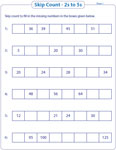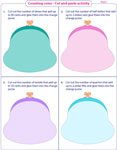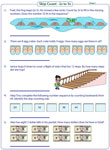Skip Counting Worksheets - Mixed Review

This module deals with skip counting from 2s to 12s. In addition there are also problems based on skip counting in 25s, 50s and 100s. The counting skills can be reviewed by number line, backward counting, word problems and many more activities. This page has more than 60 printable worksheets. Apply the skip counting rule to solve the word problems.

Number line: Type 1

These sheets have number lines based on different intervals. Fill in the missing numbers on each number line by skip counting.

2s to 5s:

6s to 12s:

2s to 10s:

Number line: Type 2

Each worksheet has two sections. Jump along the number line in intervals of 2s to 10s as shown, in the first section. In the second section, a blank number line is given. Complete each number line as per the instructions given.2s to 5s:

6s to 12s:

2s to 10s:

Skip counting: Theme worksheets

Several sheets of different themes are available here. Each worksheet has five problems and illustrated with caterpillar, fish, snail and more.2s to 5s:

6s to 12s:

2s to 10s:

Skip counting: Standard worksheets

Skip count from 2s to 100s to fill in the missing numbers.2s to 5s:

6s to 12s:

2s to 10s:

10s to 100s:

Counting with pictures: 2s to 12s

Count the items in each group to find the total. This form the basis for cumulative addition.2s to 5s:

6s to 12s:

Counting with pictures: 10s to 100s

The range of numbers is from 10 to 100. Skip count by the number given in each problem to find the total.

Cut and glue activity: 5s, 10s, 25s and 50s

Cut the given coins and glue it according to the amount mentioned in each problem. The questions are based on nickels, dimes, quarters and half dollars.Skip counting: Rule

Write the rule that describes each pattern. Fill in the missing numbers.2s to 5s:

6s to 12s:

2s to 10s:

Skip counting: Backwards

The following problems are based on skip counting from 2s to 12s. Count backwards and fill in the missing numbers.

2s to 5s:

6s to 12s:

2s to 10s:

Word Problems

Analyze and apply the skip counting rule to solve these real-life word problems.Augment skills with the entire collection of skip counting - mixed review worksheets.

Related Worksheets